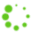# Learn STL Algorithms (C++)   Learn STL basics and algorithm

7 Sections , 50 Topics

Prerequisite

• Basic C++ Programming Knowledge
• Has written or debug some code

STL Algorithm CppCon

Standard Library Panel

Introduction to C++ and the Standard Library

C++ STL can save lot of time as well as let  us  write better code

We will be able to write code which can clearly express our intent ,Handles more Edge Cases

and are typical faster than algorithms we writeourself

Advanced topic would include creating  Apps for Mac OS Sierra/Windows

• STL Components
• Containers
• Sequence Container
• Associative Container
• Unordered Container
• Associative Arrays( Optional to know)
• Other Containers
• Iterators
• Algorithms

## Curriculum

Section  1  Non Modifying Algorithms
- C++, STL
- c++,NonModifying Algorithm
- C++, STL
- C++,STL
- C++, STL
- C++,STL
- C++,STL
- c++,STL
- c++,STL
- c++,STL
- c++,stl
12   mismatch
- c++,STL
- C++,STL
- C++,STL
- C++,STL
- C++,STL
Section  2  Modifying Algorithms
- C++,STL
19   Copy - C++,STL
20   copy_if - C++,STL
21   copy_n - c++,STL
- C++,STL
- c++,STL
- C++,STL
- c++,stl
- C++,STL
- c++,STL
28   iota - C++,STL
29   replace - C++,STL
Section  3  Removing Algorithms
- C++,STL
- C++,STL
- C++,STL
Section  4  Mutating Algorithms
- C++,STL
34   reverse - c++,STL
- C++,STL
- C++, STL
- C++,STL
- C++,STL
- C++,STL
40   Shuffle - C++,STL
Section  5  Sorting Algorithms
41   sort - C++,STL
- C++,STL
- C++,STL
Section  6  Sorted- Range Algorithms
Section  7  Numeric Algorithms